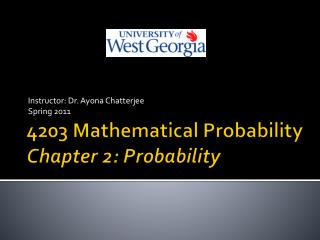DownloadDownload Presentation4203 Mathematical Probability Chapter 2: Probability

# 4203 Mathematical Probability Chapter 2: Probability

Download Presentation## 4203 Mathematical Probability Chapter 2: Probability

- - - - - - - - - - - - - - - - - - - - - - - - - - - E N D - - - - - - - - - - - - - - - - - - - - - - - - - - -
##### Presentation Transcript

1. Instructor: Dr. AyonaChatterjee Spring 2011 4203 Mathematical ProbabilityChapter 2: Probability

2. Classical Probability concept • If there are N equally likely possibilities of which one must occur and n are regarded as favorable, or as a success, then the probability of success is given by the ration n/N. • Example: Probability of drawing a red card from a pack of cards = 26/52 = 0.5.

3. Probability • Measures the likeliness of an outcome of an experiment. • The probability of an event is the proportion of the time that events of the same kind will occur in the long run. • Is a number between 0 and 1, with 0 and 1 included. • Probability of an event A is the ratio of favorable outcomes to event A to the total possible outcomes of that experiment.

4. Some Definitions • An experiment is the process by which an observation or measurement is made. • When an experiment is preformed it can result in one or more outcomes which are called events. • A sample space associated with an experiment is the set containing all possible outcomes of that experiment. It is denoted by S. • Elements of the sample space are called sample points.

5. Sample spaces • Can be • Finite (toss a coin) • Countable (toss a coin till the first head appears) • Discrete (toss a coin) • Continuous (weight of a coin)

6. Example • A manufacturer has five seemingly identical computer terminals available for shipping. Unknown to her, two of the five are defective. A particular order calls for two of the terminals and is filled by randomly selecting two of the five that are available. • List the sample space for this experiment. • Let A denote the event that the order is filled with two nondefective terminals. List the sample points in A.

7. Venn Diagram • Graphical representation of sets. • A’ is complement of event A.

8. Probability of an Event • POSTULATE 1: The probability of an event is a nonnegative real number that is P(A)>=0 for any subset A of S. • POSTULATE 2: P(S) = 1. • POSTULATE 3: If A1, A2, ….is a finite or infinite sequence of mutually exclusive events of S then P(A1 U A2 U…..)=P(A1 )+P(A2 )+……..

9. Mutually exclusive events • Two events A and B are said to be mutually exclusive if there are no elements common to the two sets. The intersection of the two sets is empty.

10. Rules of Probability • If A and A’ are complementary events in a sample space S, then P(A’)=1-P(A). Thus P(S) = 1. • P(Φ) = 0, probability of an empty set = 0. • If A and B are events in a sample space S and A is subset B the P(A) < =P(B). • If A and B are any two events in a sample space then P(A U B) = P(A)+P(B) – P(A B).

11. Conditional Probability • The conditional probability of an event A, given that an event B has occurred, is equal to • Provided P(B) > 0. • Theorem: If A and B are any two events in a sample space S and P(A)≠0, then

12. Independent Events • Two events A and B are independent if and only if • If A and B are independent then A and B’ are also independent.

13. Example • A coin is tossed three times. If A is the event that a head occurs on each of the first two tosses, B is the event that a tail occurs on the thrid toss and C is the event that exactly two tails occur in the three tosses, show that • Events A and B are independent. • Events B and C are dependent.

14. Bayes Theorem • If B1, B2, …., and Bk constitute a partition of the sample space S and P(Bi) ≠0, for i=1, 2, …., k, then for any event A in S such that P(A) ≠0

15. Reliability of a product • The reliability of a product is the probability that it will function within specified limits for a specific period of time under specified environmental conditions. • The reliability of a series system consisting of n independent components is given by • The reliability of a parallel system consisting of n independent components is given by • Ri is the reliability of the ith component.